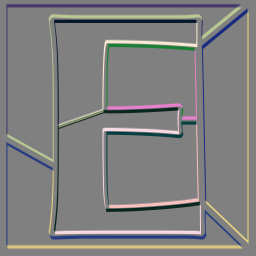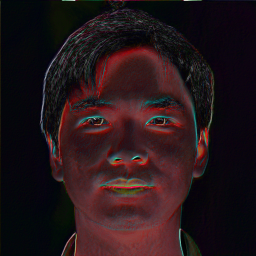G'MIC - GREYC's Magic for Image Computing: A Full-Featured Open-Source Framework for Image Processing# A Full-Featured Open-Source Framework for Image Processing

## Latest stable version: 3.2.5        Current pre-release: 3.2.6

Tutorial

Cheat #5: Vector and Pixel Access Operators

Vector Access Operator
TL;DRFuzzed E In Mathematical Expressions, right hand square brackets — V[p,q,s] — invoke a vector access operator. It returns a subvector copied from V; V is unchanged. Parameters p, q and s govern what elements copy to the subvector. Copy single elements, blocks, or elements separated by a constant stride. 1.    p sets the starting index and is required. By itself only the element indexed by p copies. 2.    q specifies how many elements in a block are copied. It is optional and defaults to one. 3.    s specifies a stride. Strides select disjoint elements separated by constant intervals. Optional and defaulting to one; the default copies contiguous elements when q > 1.
Examples:
Copy the fourth element of V into scalar SV.
\$ gmic a={V=expr('x',16);SV=V;print(V);print(SV)}
[gmic]-0./ Start G'MIC interpreter.
[gmic_math_parser] V = (uninitialized) (mem: vector16)
[gmic_math_parser] SV = (uninitialized) (mem: scalar)
[gmic_math_parser] V = [ 0,1,2,3,4,5,6,7,8,9,10,11,12,13,14,15 ] (size: 16)
[gmic_math_parser] SV = 3
[gmic]-0./ Set local variable 'a=3'.
[gmic]-0./ End G'MIC interpreter.
 1 Mathematical expression vectors are zero-indexed. With V, p is at the fourth element, counting from zero; the length of the subvector, q,  defaults to one, and the stride, s defaults to one element. 2 Unlike image lists, vector indices are non-negative. V[-1] does not select the last element of a vector V but throws an out-of-bounds exception. 3 In single-element access, G'MIC produces scalars. To explicitly obtain single-element vectors, wrap the access function in square brackets, SV=[V], which creates a one element vector from the return scalar value of V.

Copy a 3 element subvector from V starting at index=5.
\$ gmic a={V=[0,1,2,3,4,5,6,7,8,9];SV=V[5,3];print(SV)}
[gmic]-0./ Start G'MIC interpreter.
[gmic_math_parser] SV = (uninitialized) (mem: vector3)
[gmic_math_parser] SV = [ 5,6,7 ] (size: 3)
[gmic]-0./ Set local variable 'a=5,6,7'.
[gmic]-0./ End G'MIC interpreter.
 1 The length of the subvector is set to three elements. q=3. 2 The subvector receives consecutive elements starting from index=5. This stems from the default stride: s=1.

Copy a four element subvector from every fourth element in V starting at index=0.
\$ gmic a={V=[0,1,2,3,4,5,6,7,8,9,10,11,12,13,14,15];SV=V[0,4,4];print(SV)}
[gmic]-0./ Start G'MIC interpreter.
[gmic_math_parser] SV = (uninitialized) (mem: vector4)
[gmic_math_parser] SV = [ 0,4,8,12 ] (size: 4)
[gmic]-0./ Set local variable 'a=0,4,8,12'.
[gmic]-0./ End G'MIC interpreter.
 1 The length of the subvector is set to four elements. q=4. 2 The stride of the operation, s=4, copies disjoint elements separated from each other by s-1 intervening elements.

Join six elements from V situated at even indices, starting at index=0, with five disjoint elements, also from V, starting at index=3 and separated by two intervening elements.
\$ gmic a='{V=[0,1,2,3,4,5,6,7,8,9,10,11,12,13,14,15];[V[0,6,2],V[3,5,3]]}'
[gmic]-0./ Start G'MIC interpreter.
[gmic]-0./ Set local variable 'a=0,2,4,6,8,10,3,6,9,12,15'.
[gmic]-0./ End G'MIC interpreter.
 1 Square brackets not otherwise decorating existing vectors create new ones.  Encompassing a list with square brackets creates a vector with a length equal to the length of the list. List elements themselves may be vectors, as seen here. The operation concatenates the vectors in the order that they appear.
Setting Elements
When on the left hand side of an assignment, a vector accessor operator permits only one element assignment. Attempting to set multiple elements of a left hand vector with q>1 or s>1 raises an exception.
\$ gmic a={V=expr('x',20);V[0,5,5]=320;print(V)}
[gmic]-0./ Start G'MIC interpreter.
[gmic]-0./ *** Error *** Item substitution '{V=expr('x',20);V[0,5,5]=3;print(V)}': Unrecognized item '0,5,5' in expression 'V[0,5,5]=3'.

However, with q==1 and s==1, the defaults, the single element addressed by p can be altered through assignment.
\$ gmic a={V=expr('x',20);V=320;print(V)}
[gmic]-0./ Start G'MIC interpreter.
[gmic_math_parser] V = (uninitialized) (mem: vector20)
[gmic_math_parser] V = [ 0,1,2,3,4,320,6,7,8,9,10,11,12,13,14,15,16,17,18,19 ] (size: 20)
[gmic]-0./ Set local variable 'a=0,1,2,3,4,320,6,7,8,9,10,11,12,13,14,15,16,17,18,19'.
[gmic]-0./ End G'MIC interpreter.
Pixel Access Operators
TL;DR
 Pixel Access Operators obtain p ixel el ements, pels, or pixels — a column vector of pels, and are the core "setters" and "getters" for pel and pixel processors, which are the math expressions serving as kernels for iterations mediated by fill, eval or input. In their simplest configurations, sans arguments, pixel access operators can set or get the current pel or pixel, operating on both sides of an assignment. More detailed schemes arise from writing operators with specific styles of argument brackets, identifying letters and letter cases. A tabulation of such styles follows.
Examples:face average face
Replace every pixel by the average of its channel values.
\$gmic images/dne_face.png -fill. ip=sum(I)/s;vectors(ip)
[gmic]-0./ Start G'MIC interpreter.
[gmic]-0./ Input file 'images/dne_face.png' at position 0 (1 image 1024x1024x1x3).
[gmic]-1./ Fill image  with expression 'ip=sum(I)/s;vectors(ip)'.
[gmic]-1./ Display image  = 'dne_face.png'.
 = 'images/dne_face.png':
size = (1024,1024,1,3) [12 Mio of float32].
data = (186.667,190,193,195.667,197,197,195.667,195,195,195,196.667,195.667,(...),189.333,189.667,190.667,189.667,190.667,191.333,191.333,191.333,191.333,191.333,190.333,186).
min = 13.3333, max = 225, mean = 162.022, std = 49.5293, coords_min = (581,136,0,0), coords_max = (460,0,0,0).
 1 The pixel access operator appears here in a vector form: I. In the absence of explicit arguments, the immediate value of I varies as the current location changes under iterations mediated by fill, input or eval. "Vector form" means that I provides the entire pixel at the current location as column vector of length s, the depth of the current image. 2 The aim of writing vectors(ip), and not an unadorned ip, is to obtain a pixel processor; these iterate over images a pixel-at-a-time and are called into play by fashioning processors with vector return values. The unadorned ip is scalar, the return type of sum(). Unaltered, this scalar return value would have fashioned a pel processor. These iterate over images a pel at-a-time, the finer granularity requiring traversal over individual image planes — three times as many steps with an RGB image. This finer-grained traversal happens to achieve the same result but at unnecessary cost. 3 Terse notation bears careful reading. At first glance, vectors(ip) suggests a plural form of vector(). But recall that this constructor has a compound form: "vector" plus "number" — or what can resolve to a number. So vector8(0) returns an eight element vector populated with zeros; vectors(3.5) returns a vector whose length equals the depth of the current image (s) and with all elements set to 3.5.face diff face
Take the L2 norm of every delta between a pel and a neighbor offset in position by [-3,-3,0,-1].
\$ gmic -input images/dne_face.png -fill. 'norm2(i-j(-3,-3,0,-1,0,2))'
[gmic]-0./ Start G'MIC interpreter.
[gmic]-0./ Input file 'images/dne_face.png' at position 0 (1 image 1024x1024x1x3).
[gmic]-1./ Fill image  with expression 'norm2(i-j(-3,-3,0,-1,0,2))'.
[gmic]-1./ Display image  = 'dne_face.png'.
 = 'images/dne_face.png':
size = (1024,1024,1,3) [12 Mio of float32].
data = (1,5,10,3,4,4,2,2,1,0,2,0,(...),11,9,8,11,11,10,10,10,9,8,9,13).
min = 0, max = 168, mean = 16.2062, std = 17.0729, coords_min = (9,0,0,0), coords_max = (298,0,0,1).
[gmic]-1./ End G'MIC interpreter.
 1 The pixel access operator appears in two scalar forms: i, and j(-3,-3,0,-1,0,2). In pel processors i, sans arguments, references the channel value at the current location; it varies with changing location throughout the iteration, taking on the pel value of the current position. j(-3,-3,0,-1,0,2) accesses the pel offset from the current location in the direction [-3,-3,0,-1]. The L2 norm of their difference measures a color space distance. A large color space distance — from red to cyan, say — signals an edge in the image space direction (-3, -3, 0, -1). 2 The fifth and sixth arguments to j(…0,2) set interpolation and boundary policies. Interpolation puts forward the idea that image pixels are sample points and it is reasonable to access values between them. In such cases, the fifth parameter, an interpolation flag 1, provides for linear interpolation, while 2 provides for cubic interpolation. 0, used here, establishes discrete sampling. There are no "in-between" values; sampling assumes the value of the nearest pel or pixel. 3 In the matter of returning results, mathematical expressions given to pel and pixel processors as arguments to input and fill behave differently  from eval. In the first two, the value of the last statement in the expression sets the pel or pixel value at the current location; an implied assignment. input and fill processors "paint" the image. eval leaves the pel or pixel unchanged — no implicit assignment. It "evaluates" the image without (necessarily) changing it, though indirect changes might stem from other statements in the processor.

A Pixel Access Operator Menagerie
The foregoing examples illustrate the diverse notation exhibited by pixel access operators. Select behavior through choice of (1) operator names (2) argument brackets, or (3) letter case.

 i/j v. I/J Pel\Pixel Lower-case operator names, i and j, return scalar pels. Necessarily, fully addressing specific pels requires coordinates in width, x, height, y, depth, z and channel size c. Upper-case operator names, I and J return vector pixels. This coaser-grained addressing scheme dispenses with channel addressing, as the pixel slices through all channels. i/I v. j/J Origin\current location With operator names i or I, Coordinate arguments x, y, z and c measure from the image origin. With j or J operator names, measures are relative to the current location. [] v. () Vector\Image Square brackets around the arguments address image elements as if occupying a one dimensional vector and gives rise to a modified signature: [#ind,i,boundary_conditions]. The one index, i, measures from the start of this vector (i/I) or from the current location mapped into the vector (j/J), the index furnishing an offset. During iterations, the lower-case scalar form reads out memory storage: first the red channel, then green, then blue, presuming an RGB image. Upper-case I/J vectors stride across image channels and assemble pixels. The square bracket form does not support an interpolation argument, but does take a boundary_condition flag to handle off-image access. Parenthetically bracketed arguments give rise to the image-oriented signature: (#ind,x,y,z,c,interpolation,boundary_condition). This signature is for lower-case pel operators, i/j which work with a high-resolution view of image contents, locating a specific pel by image column, x, image row y, slice, z and channel c; see Images. Omitting the channel argument c gives rise to the upper-case pixel operators I/J. These work with a lower resolution view of the image contents and return entire pixels at each call: column vectors with length s, the spectrum, or channel count, of the image. With both signatures, a numeric first argument with a preceding # signet is taken to be the index to an image on the list index. Operations then proceed on that image instead of the one associated with the math expression. In the absence of explicit association, it is the last image on the list. Also with both signatures, a first argument of the form #\$__, then operations proceed on the named image. Here, a recurring theme: terse notation bears careful reading. For the (1) uninitiated, (2) sleep and/or coffee deprived, or (3) generally inattentive, the day will come when the pixel access functions i[…], I[…], j[…] or J[…] will confusticate with vector access right hand decorators [] and the afflicted coder will write I[p,q,r] vector access argument signatures where pixel access signatures were intended. The effect is not fatal; it will access neither pixels nor vectors but raise exceptions instead.
Fuzz E
The frontpiece image toy furnishes an extended play on differencing neighboring pixels for what may be construed as a chiseling in stone effect.
fuzze: -check \${1=1.5}>0" && "\
isnum(\${1})" && "\
isnum(\${2=45})" && "\
\${2}>=0" && "\
\${2}<=180
-skip \${3=8}
-if s<3
-to_rgb.
-fi
fz={0.1*\$1}
dpth=\$3
isa={!(s%2)}
-fill. ">
OFS=cexp([\$fz,\$ang]);
V=I-J(OFS,OFS,0,2,2);
\$isa?V[s-1]=2^\$dpth-1:V;
V
"
-shared. 0,{s-(1+\$isa)}
-normalize. 0,{2^\$dpth-1}
-remove.
Command line:
Note last argument to fuzze. .png and .tiff image formats support various bit resolutions: 8, 16 and 32 being common. input can detect this resolution and return it in status, \${} as a numeric: 8, 16, 32… If detection fails, the return status \${} contains an empty string.
gmic fuzze.gmic -input images/e_letter.png fuzze. 12.5,72,\${} -output. img/fuzze.png

Updated: March 7 2023 12:15 UTC Commit 220bd6b91cf3556158551b6ca6d8f6e242b72850
G'MIC - GREYC's Magic for Image Computing: A Full-Featured Open-Source Framework for Image Processing